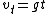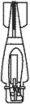free fall

(redirected from free-falls)
Also found in: Dictionary, Thesaurus.

free fall,

in physics, the state of a body moving solely under the influence of gravitational forces (see gravitationgravitation,
the attractive force existing between any two particles of matter. The Law of Universal Gravitation

Since the gravitational force is experienced by all matter in the universe, from the largest galaxies down to the smallest particles, it is often called
). A body falling freely toward the surface of the earth undergoes an accelerationacceleration,
change in the velocity of a body with respect to time. Since velocity is a vector quantity, involving both magnitude and direction, acceleration is also a vector. In order to produce an acceleration, a force must be applied to the body.
due to gravity of 32 ft/sec2 (9.8 m/sec2), which is symbolized by g.

Free fall

The accelerated motion toward the center of the Earth of a body acted on by the Earth's gravitational attraction and by no other force. If a body falls freely from rest near the surface of the Earth, it gains a velocity of approximately 9.8 m/s every second. Thus, the acceleration of gravity g equals 9.8 m/s2 or 32.16 ft/s2. This acceleration is independent of the mass or nature of the falling body. For short distances of free fall, the value of g may be considered constant. After t seconds the velocity vt of a body failing from rest near the Earth is given by Eq. (1).

(1)If a falling body has an initial constant velocity in any direction, it retains that velocity if no other forces are present. If other forces are present, they may change the observed direction and rate of fall of the body, but they do not change the Earth's gravitational pull; therefore a body may still be thought of as freely “failing’’ even though the resultant observed motion is upward.

For a body failing a very large distance from the Earth, the acceleration of gravity can no longer be considered constant. According to Newton's law of gravitation, the force between any two bodies varies inversely with the square of the distance between them; therefore with increasing distance between any body and the Earth, the acceleration of the body toward the Earth decreases rapidly. The final velocity vf, attained when a body falls freely from an infinite distance to the surface of the Earth, is given by Eq. (2),

(2)where R is the radius of the Earth, which gives a numerical value of 11.3 km/s or 7 mi/s. This is consequently the “escape velocity,’’ the initial upward velocity for a rising body to completely overcome the Earth's attraction.

Because of the independent action of the forces involved, a ball thrown horizontally or a projectile fired horizontally with velocity v will be accelerated downward at the same rate as a body falling from rest, regardless of the horizontal motion.

At a sufficiently large horizontal velocity, a projectile would fall from the horizontal only at the same rate that the surface of the Earth curves away beneath it. The projectile would thus remain at the same elevation above the Earth and in effect become an Earth satellite. See Ballistics, Gravitation

free fall

Motion of a body under the influence of gravity alone, i.e. with no other forces acting. See also weightlessness.

free fall

[′frē ‚fȯl]
(mechanics)
The ideal falling motion of a body acted upon only by the pull of the earth's gravitational field.
(petroleum engineering)
In deep drilling, an arrangement by which the bit is permitted to fall freely to the bottom at each drop or down stroke.

free fall

1. The descent of freshly mixed concrete into forms without dropchutes or other means of confinement.
2. The distance through which such descent occurs.
3. The uncontrolled fall of aggregate.

free fallA conventional free-fall bomb.Laser-guided bomb.
i. Any jump in which the parachutist pulls his own ripcord.
ii. The fall or drop of a body, such as a rocket, not guided, not under thrust, and not retarded by a parachute or other braking device. free-fall bomb An unguided bomb that follows the rules of ballistics.

free fall

1. free descent of a body in which the gravitational force is the only force acting on it
2. the part of a parachute descent before the parachute opens
Site: Follow: Share:
Open / Close